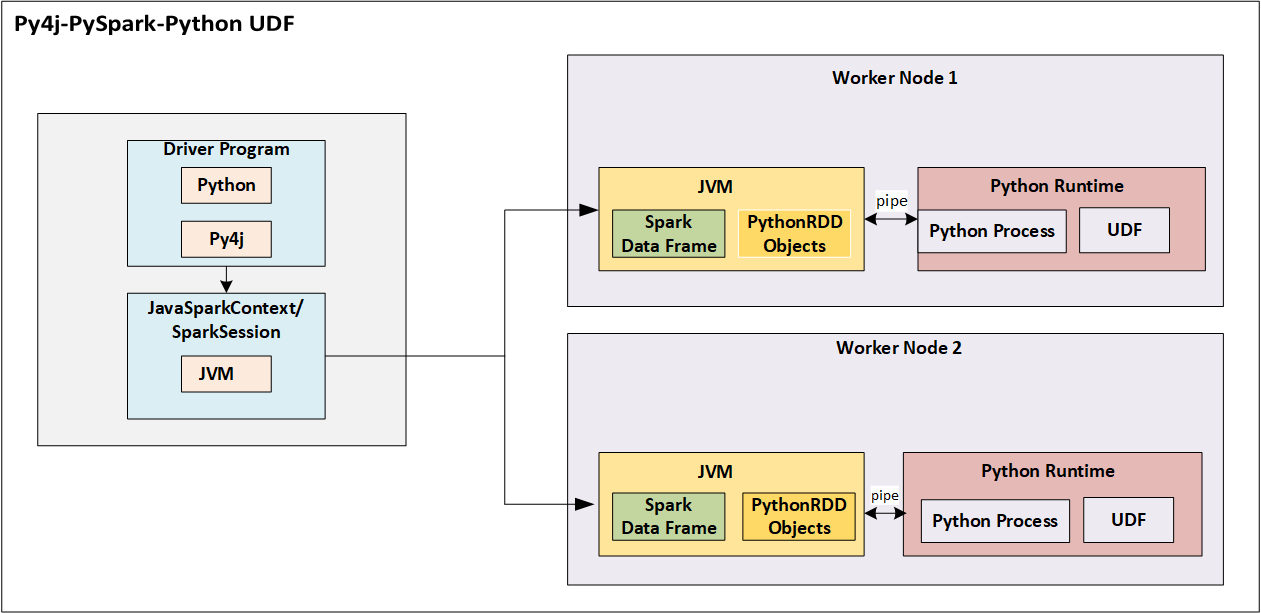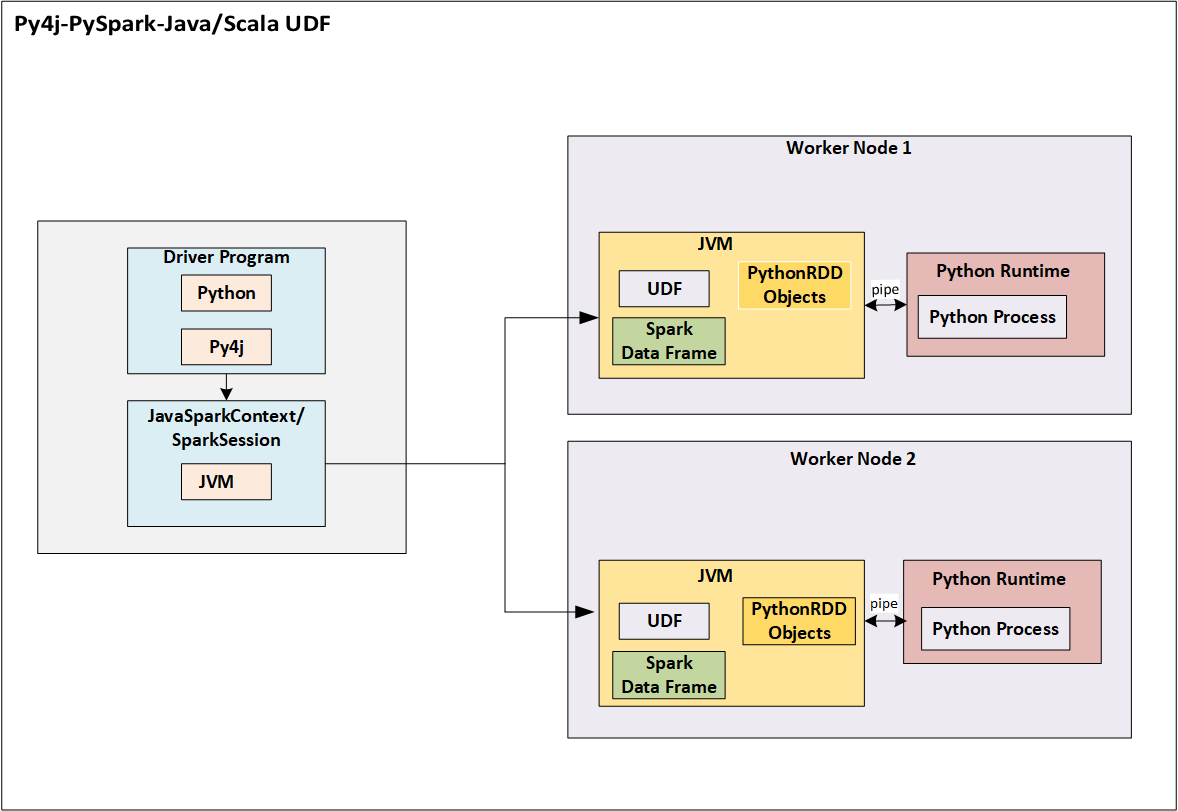{{announcement.body}}
{{announcement.title}}

# PySpark: Java UDF Integration

DZone 's Guide to

# PySpark: Java UDF Integration

· Integration Zone ·
Free Resource

Comment (0)

Save
{{ articles.views | formatCount}} Views

PySpark is the Spark API implementation using the Non-JVM language Python. Though developers utilize PySpark by implementing Python Code using Spark API’s (Python version of Spark API’s), internally, Spark uses data to be cached in JVM.

The Python Driver Program has SparkContext, which uses Py4J, a specialized library for Python Java interoperability to launch JVM and create a JavaSparkContext.

On a high level, transformations on RDD in Python are mapped to transformations on PythonRDD objects in Java.

PythonRDD objects in the Executors launch Python subprocess, which communicates using pipes (internally uses Sockets) that transfers data and code that undergoes serialization and deserialization process

In order to remove this overhead of serialization and deserialization, we need to leverage Spark DataFrames in PySpark where the data remains inside the JVM as long as possible rather than going for RDD’s.

User Defined Functions are used in Spark SQL for custom transformations of data, which are very useful if Spark built-in transformations are not supported for any business rule to be implemented

If any user-defined function is implemented in Python, internally this undergoes data to be serialized from JVM and be passed into separate Python process where UDF runs. The result of UDF execution is passed further back to serialization, deserialization and returned to JVM.

This incurs a lot of performance overhead as this is undergoing Serialization and Deserialization in 2 trips.Fig 1.UDF implementation in Python where this function is utilized in PySpark SQL implementation

To avoid the performance overhead, the recommendation is to implement UDF in Java/Scala that will be utilizing JVM itself as the environment for execution, which eliminates the need for serialization and network tripFig 2.UDF implementation in Java where this function is utilized in PySpark SQL implementation

I would be showcasing a proof of concept that integrates Java UDF in PySpark code

Implement 2 classes in Java that implements org.apache.spark.sql.api.java.UDF1 interface

``````package com.JavaUDFProj;
import org.apache.spark.sql.api.java.UDF1;
public class AddNumber implements UDF1<Long, Long> {
private static final long serialVersionUID = 1L;
@Override
public Long call(Long num) throws Exception {
return (num + 5);
}
}``````

AddNumber.java takes the variable of the long data type as the input argument, adds 5 to this variable, and gives the result

Another class MultiplyNumber.java takes the variable of the long data type as the input argument, multiplies by 5 to this variable, and gives the result

``````package com.JavaUDFProj;
import org.apache.spark.sql.api.java.UDF1;
public class MultiplyNumber implements UDF1<Long, Long> {
private static final long serialVersionUID = 1L;
@Override
public Long call(Long num) throws Exception {
return (num * 5);
}
}``````

The Maven dependency to these 2 UDF1 implementation classes is as below:

``````<dependency>
<groupId>org.apache.spark</groupId>
<artifactId>spark-core_2.11</artifactId>
<version>2.0.0</version>
</dependency>
<dependency>
<groupId>org.apache.spark</groupId>
<artifactId>spark-sql_2.11</artifactId>
<version>2.0.0</version>
</dependency>``````

Compile the above 2 Java classes and make it as one jar, which is named in this example as “javaudfdemo.jar”.

PySpark code is tested with Spark 2.3.1 version

The PySpark code is as below:

``````import pyspark
from pyspark.sql import SparkSession
from pyspark.sql.types import LongType
from pyspark import SparkContext
sc = SparkContext()
spark = SparkSession.builder.config("spark.sql.warehouse.dir", "file:///c:/tmp/spark-warehouse").getOrCreate()
spark.udf.registerJavaFunction("numMultiply", "com.JavaUDFProj.MultiplyNumber", LongType())
import json
j = {'num1':2, 'num2':3}
a=[json.dumps(j)]
jsonRDD = sc.parallelize(a)
df.registerTempTable("numbersdata")
df1=spark.sql("SELECT numMultiply(num1) As num1, numAdd(num2) AS num2 from numbersdata")
df1.show(10)``````

In PySpark, register the 2 Java classes as given below

spark.udf.registerJavaFunction("numMultiply", "com.JavaUDFProj.MultiplyNumber", LongType())

registerJavaFunction takes 3 arguments, which are function name to be used in spark sql, Java class name that implements UDF and the return type of UDF. The registerJavaFunction will register UDF to be used in Spark SQL.

In this example, PySpark code, JSON is given as input, which is further created as a DataFrame.

A SQL query is issued on the DataFrame registered as a table with the name “numbersdata” and the columns are transformed using the UDF’s.

In this example, the transformation functions are UDF's that registered with names "numMultiply" and "numAdd"

Run the PySpark command using as below command from the windows command prompt or shell.

Issue spark-submit command in the folder, in this example, the jar and Python files are present in the same location that spark-submit is invoked.

spark-submit –jars javaudfdemo.jar test.py

The output is as below:

+----+----+

|num1|num2|

+----+----+

|  10|   8|

+----+----+

In this way, user-defined functions implemented in Java can be called from PySpark, which will improve the performance of the application rather than implementing functions in Python.

Topics:
api implementation, integration, java, pyspark, python, serialization, spark, tutorial, udf integration, user defined functions

Comment (0)

Save
{{ articles.views | formatCount}} Views

Opinions expressed by DZone contributors are their own.